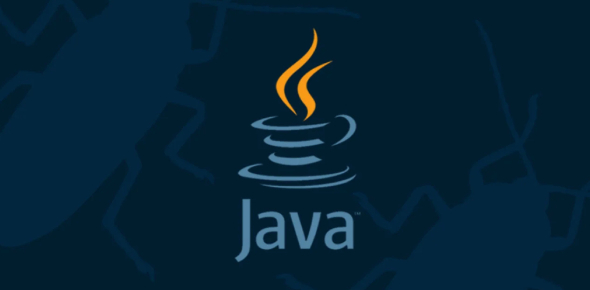# Quiz: The Ultimate Java Test For Beginners

93 Questions | Attempts: 25810
ShareSettingsThis is the ultimate Java test for all those Java developer-wannabes who have just began practicing the programming language. It consists of over 93 questions of basically Core Java. So, if you are one of them and want to have a solid practice session to strengthen your hold on the concepts and methods, take it right now.

• 1.
Object-Oriented Programming means ...
• A.

Being objective about what you develop

• B.

Designing the application based on the objects discovered when analysing the problem

• C.

Writing an algorithm before writing your program and having a test plan

• D.

Writing a program composed of Java classes

• 2.
If none of the private/protected/public is specified for a member, that member ...
• A.

Is accessible publicly

• B.

Is only accessible by other classes of the same package

• C.

Is only accessible from within the class

• D.

Is accessible by the class and its subclasses

• 3.
Which one is not correct?
• A.

A class needs to be instantiated as an object before being used

• B.

An objects exists in memory in run time

• C.

Class and object are just different names for the same thing

• D.

An object is a variable, where its type is the class used to declare the variable

• 4.
Which is not a part of defining an object?
• A.

Description

• B.

Methods

• C.

Associations with other objects

• D.

Name

• 5.
Class B inherits from Class A, what cannot be said:
• A.

B is a sub-class of A

• B.

A is a super-class of B

• C.

• D.

• 6.
What is a member of a class
• A.

An attribute

• B.

A method

• C.

Attribute or method

• D.

A sub-class

• 7.
A UML association from class A to class B means
• A.

Class B inherits from Class A

• B.

Class B is defined within class A

• C.

Class B has an attribute of type A

• D.

Class A has an attribute of type B

• 8.
A UML association is ...
• A.

Implemented as a Java attribute member

• B.

Implemented as a Java method

• C.

Implemented as a sub-class

• D.

Implemented as a String constructor returning the name of the association target

• 9.
An object could be ...
• A.

Anything

• B.

An algorithm

• C.

A data container

• D.

A program

• 10.
A class is...
• A.

An object

• B.

An executable piece of code

• C.

An abstract definition of an object

• D.

A varibale

• 11.
The size of a frame is set using ...
• A.

The method setSize()

• B.

Automtically in run-time

• C.

The width and height attributes of the class JFrame

• D.

WIDTH and HEIGHT properties of the window menu in Eclipse

• 12.
Which package do you need to use widgets such as JApplet, JFrame, JPanel and JButton?
• A.

Javax.swing

• B.

Javax.gui

• C.

Java.awt

• D.

Java.swing

• 13.
Which one needs a web page to run
• A.

A Java Application

• B.

A Java Stand-Alone Application

• C.

A Java Applet

• D.

A Java Class

• 14.
What does GUI stand for?
• A.

Graphical User Interface

• B.

Gimme Ur Internet

• C.

Grand User Interface

• D.

Graphical Useful Interface

• 15.
How is the layout of widgets on a panel specified?
• A.

Automatically

• B.

By inheriting from the main container such as a JFrame or JApplet

• C.

By calling the method setLayout

• D.

By calling the method setContentPane

• 16.
Which one adds the widget mainPanel to a frame in the constructor of the frame?
• A.

• B.

• C.

MainPanel.setVisible(true);

• D.

• 17.
Which one could be used as the main container in a Java application?
• A.

JApplet

• B.

JFrame

• C.

JPanel

• D.

JButton

• 18.
How to define a JButton with the caption test?
• A.

JButton aButton('test');

• B.

JButton aButton=new JButton("test");

• C.

JButton aButton=new JButton('test');

• D.

JButton("test") aButton;

• 19.
Which one adds the widget mainPanel to an applet in the init method of the applet?
• A.

• B.

• C.

MainPanel.setVisible(true);

• D.

• 20.
The size of an applet is set using ...
• A.

The method setSize()

• B.

Automtically in browser

• C.

The width and height attributes of the class JApplet

• D.

HTML properties WIDTH and HEIGHT of the APPLET tag.

• 21.
The last value in an array called ar can be found at index:
• A.

0

• B.

1

• C.

Ar.length

• D.

Ar.length - 1

• 22.
What would display from the following statements? int [ ] nums = {1,2,3,4,5,6}; System.out.println((nums + nums));
• A.

6

• B.

2+4

• C.

1+3

• D.

4

• 23.
What loop will display each of the numbers in this array on a separate line: float [ ] nums= {1.1f, 2.2f, 3.3f};
• A.

For (int i =0; i < 3; i++) System.out.println( nums[i]);

• B.

For (i = 1; i

• C.

For (i = 0; i

• D.

For (i = 1; i < 3; i++) System.out.println(nums[i]);

• 24.
What displays from the following statements? String word = "abcde"; for (int i = 0; i <4; i+=2) System.out.print(word[i]);
• A.

Ab

• B.

Ac

• C.

Ace

• D.

Bd

• 25.
Given the declaration : int [ ] ar = {1,2,3,4,5}; What is the value of ar?
• A.

2

• B.

3

• C.

4

• D.

5

## Related TopicsBack to top
×

Wait!
Here's an interesting quiz for you.